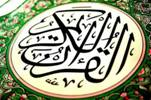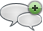### Special numbers of Quran

#### الأربعاء، 22 يوليو 2015 - 0:36The Quran Book has 114 chapters. So N=114 Pivot chapter (Al-7adeed) = N/2 = 57 The pivot chapter is a multiplication of two prime numbers P1*P2=3*19 Number of Pivot chapter verses is prime number P3 = median (1..N/2) = 29 The product of P1*P2*P3 = 3*19*29 = 1653 has the property where the 16th prime = 53أضف تعليقك
##### رجاء ضرورة الإلتزام بعدم إضافة أي تعليق يمس أو يسيء للأديان أو المعتقدات أو المقدسات, كما نرجو ألا يتضمن التعليق السباب أو أي ألفاظ تخدش الحياء والذوق العام

 الأكثر قراءةالسجدة.Chapters Whose Number is the Square of a Prime Number.تعيين ليلة القدر من سورة الدخان.تعيين ليلة القدر.نوح عليه السلام.Number 114 is an Indication of Completeness.Sura Alfati7a, Alfalaq and Annas all use 21 Letters of the Alphabet.333667.لطائف للرقم 7 في سورة الفاتحة.حساب الجمّل علم الأنبياء.
الموقع الرسمي حم © 2015, كل الحقوق محفوظة |